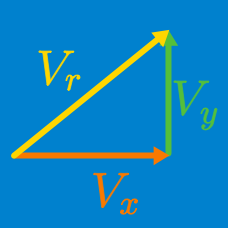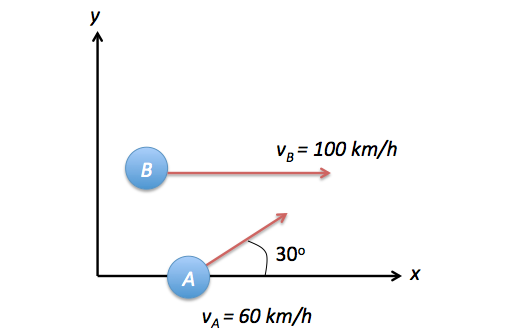Classical Mechanics

# Vector Kinematics

#### Challenge Quizzes

The distance from Boston to San Francisco is 4344 km. However, a flight from Boston to San Francisco is about 6.33 hours of flying time (the flying time is the total time minus the time required to takeoff and land), whereas a flight from San Francisco to Boston is only 5.33 hours of flying time.

The difference is due to the jet stream, which blows across the United States roughly from west to east. If the speed of the airliner relative to the air is a constant, and the jet stream is considered as blowing at a constant speed directly against the airplane as it flies from Boston to San Francisco and directly with the airplane on the return flight, then what is the speed of the jet stream in km/hour?Snow is falling vertically at a constant speed of $8.0 \text{ m/s.}$ At what angle from the vertical do the snowflakes appear to be falling as viewed by the driver of a car traveling on a straight, level road with a speed of $50 \text{ km/h?}$

A train is moving at $21\text{ km/h}.$ If a passenger on the train runs towards the front of train at $1\text{ km/h},$ what is the relative velocity of outside trees in terms of that passenger?

A plane can propel itself at a speed of $124 \text{ km/hr} .$ If the plane encounters a $93 \text{ km/hr}$ crosswind, what is the resultant speed of the plane?Particle $A$ moves at a speed of $60 \text{ km/h}$ in a direction that makes a $30 ^{\circ}$ angle with the $x \text{-axis} ,$ and particle $B$ moves at $100 \text{ km/h}$ in the direction of the $x \text{-axis}.$ Determine the approximate relative velocity of particle $B$ with respect to particle $A.$

×